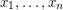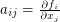# Jacobian Conjecture

 Importance: High ✭✭✭
 Author(s): Keller, Otto-Heinrich
 Subject: Geometry » Algebraic Geometry
 Keywords: Affine Geometry Automorphisms Polynomials
 Posted by: Charles on: July 6th, 2008
Conjecture   Letbe a field of characteristic zero. A collectionof polynomials in variablesdefines an automorphism ofif and only if the Jacobian matrix is a nonzero constant.

The Jacobian determinant is the determinant of the matrixwith. It is elementary to show that if the mapis an automorphism, then the Jacobian determinant is a nonzero constant, by using the inverse map. The other direction has turned out to be rather difficult.

It is known that the Conjecture holds for polynomials of degree 2, and that the general case follows from a special case in degree 3.

## Bibliography

* indicates original appearance(s) of problem.

### Problem statement

iff the determinant of the Jacobian matrix is a nonzero constant.